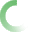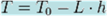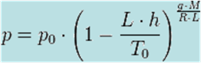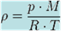﻿ Air Density Calculations Impact of Altitude Temperature Humidity and Pressure.xlsxAir Density Calculator Altitude, Temperature, Humidity and Barometric Pressure of Weather Systems and their impact on local atmospheric air density. For accurate measurement of local air conditions we recommend a high accuracy & robust logging weather station such as the ones sold on BARANI DESIGN weather station shop and allMETEO.com live streaming weather portal. AIR DENSITY CALCULATION INPUTS (local atmospheric conditions) Property Value Unit of Measure Alternate Units Description / Note Air Temperature = C (degrees) F (degrees) Local air temperature Air Humidity = % (Rel. Humidity) g/m3 (ABS Humidity) Relative and corresponding Absolute Humidity value. *Barometric Pressure = hPa (hectoPascals) mmHg (mm of Mercury) *If pressure value is set to "0", Altitude value is used to calculate local barometric pressure based on the 1976 Standard Atmosphere Model. Altitude / Elevation = meters ft Altitude above Sea Level /elevation AIR DENSITY CALCULATOR OUTPUT **Air Density = kg/m3 lb/ft3 Local air density corrected for local altitude, temperature, humidity and barometric pressure. **Local air density is a very important factor in determinimg wind turbine performance and engine horse power output. Each factor like altitude above sea level, air temperature, humidity, and even barometric pressure due to local weather systems can have significant effect on local air density. **Thus, if one wants to accurately predict wind turbine performance, it is not only important to use an accurate anemometer to measure wind speed, but to also measure all the other atmospheric factors which can have up to 10% influence on wind energy calculations. ***Dew Point Air Temperature = C (degrees) F (degrees) Air temperature at which air humidity starts to condense into water droplets. ***If an object at this temperature is located in the air environment of the above given conditions, the object, like a car window, will fog up due to air humidity condensing on its surface. This calculator is brought to you by? = kg/m3 air density corrected for altitude, temperature and pressure ?_corrected = kg/m3 air density corrected for altitude, temperature, pressure and humidity. D = density, kg/m3 Pd = pressure of dry air (partial pressure), Pascals Pv= pressure of water vapor (partial pressure), Pascals P = Pd + Pv = total air pressure, Pascals ( multiply mb by 100 to get Pascals) Rd = 287.05 gas constant for dry air, J/(kg*degK) = 287.05 = R/Md Rv = 461.495 gas constant for water vapor, J/(kg*degK) = 461.495 = R/Mv R = 8314.32 universal gas constant = 8314.32 (in 1976 Standard Atmosphere) Md = 28.964 molecular weight of dry air = 28.964 gm/mol Mv = 18.016 molecular weight of water vapor = 18.016 gm/mol T = temperature, deg K = deg C + 273.15 Standard Atmosphere p0= 101325 Pa T0= 288.15 K ?0= 1.225 kg/m³ To calculate the density of air as a function of altitude, one requires additional parameters. They are listed below, along with their values according to the International Standard Atmosphere, using the universal gas constant instead of the specific one: Temperature at altitude h meters above sea level is given by the following formula (only valid inside the troposphere): T standard = KT Non-standard = Kpressure at altitude g*M/R/L = p = Padensity can then be calculated according to this molar form formula ? = kg/m3 ?_non_std_temp = kg/m3 Temp = C ambient temperature h = meters Altitude above sea level p0 = 101325 Pa sea level standard atmospheric pressure T0 = 288.15 K sea level standard temperature g = 9.80665 m/s2 Earth-surface gravitational acceleration L = 0.0065 K/m temperature lapse rate R = 8.31447 J/(mol·K) universal gas constant M = 0.0289644 kg/mol molar mass of dry air Es = Pascals (Pa)saturation pressure of water vapor Tc = temperature, deg C c0 = 6.1078 c1 = 7.5 c2 = 237.3 Pv = Rh*Es Pv = actual water vapor pressure Es = RH % = P @ Alt & Temp = Pascals (Pa) actual water vapor pressure P = Pascals (Pa) absolute atmospheric pressure h = meters altitude above Sea Level Density correction for humidity multiplier = ratio td = 243.12*H/(17.62-H) °C H = (log10(RH)-2.0)/0.4343+(17.62*T)/(243.12+T) td = °C dew Point Temperature H = RH % = Temp = m = 17.62 Tn = 243.12 °C A = 6.112 hPa dv = g/m3absolute HumidityCopyright BARANI DESIGN Technologies © 2012